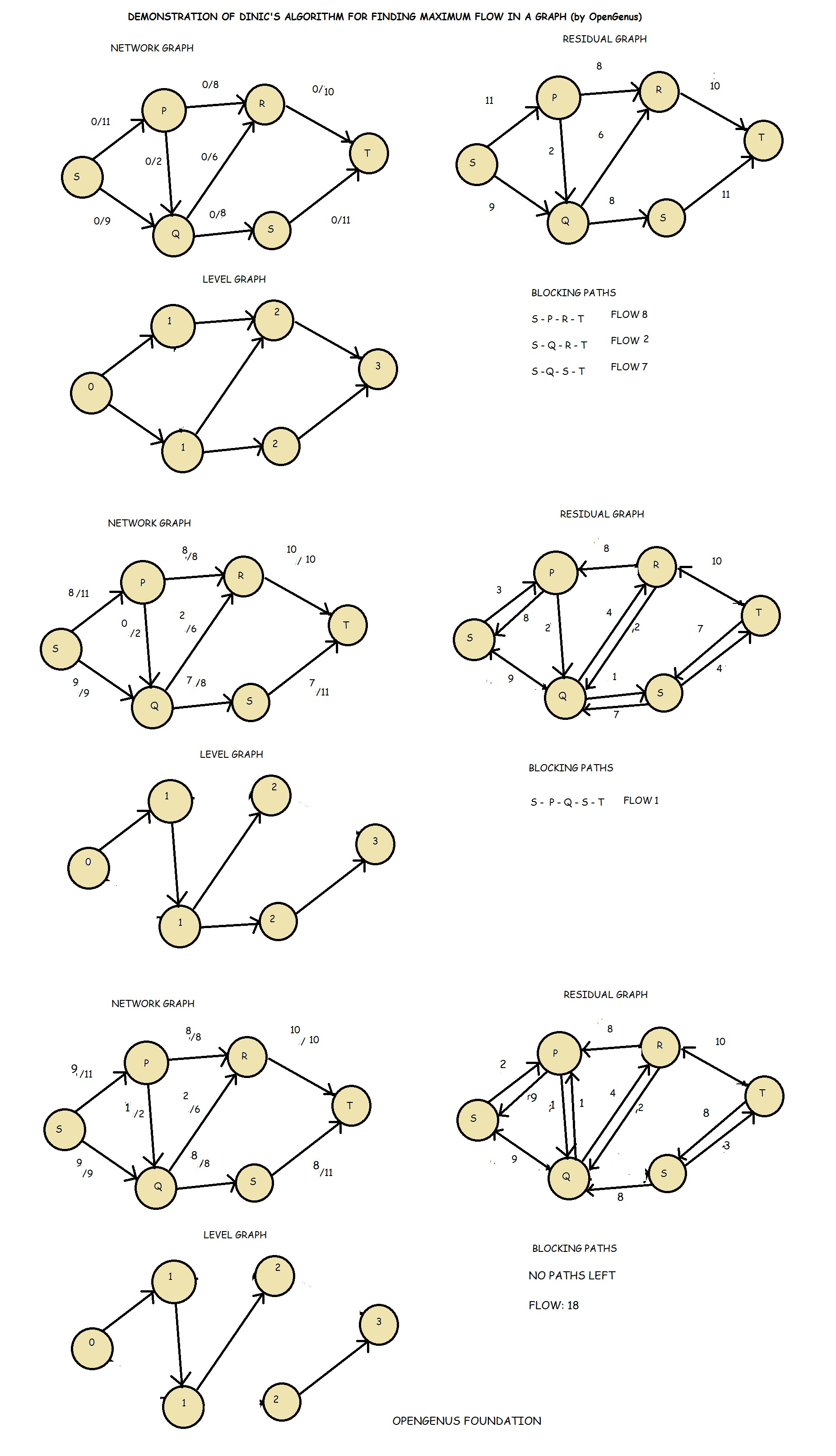×

Search anything:

# Dinic's algorithm for Maximum flow in a graph

#### Graph Algorithms maximum flow dinic's algorithmGet this book -> Problems on Array: For Interviews and Competitive Programming

Reading time: 15 minutes | Coding time: 9 minutes

Dinic's algorithm or Dinitz's algorithm is a strongly polynomial algorithm for computing the maximum flow in a flow network. The basic principle is that a Maximum flow = minimum cut and Breadth First Search is used as a sub-routine.

The credit of Dinic's algorithm goes to computer scientist Yefim (Chaim) A. Dinitz.

### Pseudocode

``````
1.set f(e) = 0 for each e in E
2.Construct G_L from G_f of G. if dist(t) == inf, then stop and output f
3.Find a blocking flow fp in G_L
4.Augment flow f by fp  and go back to step 2.
``````

Level graph is one where value of each node is its shortest distance from source.

Blocking flow includes finding the new path from the bottleneck node.

An augmenting path is a simple path from source to sink which do not include any cycles and that pass only through positive weighted edges. A residual network graph indicates how much more flow is allowed in each edge in the network graph. If there are no augmenting paths possible from to , then the flow is maximum.

Follow the following illustrative example of Dinic's algorithm:### Complexity

• Worst case time complexity: `Θ(E * V * V)`
• Average case time complexity: `Θ(E * V * V)`
• Best case time complexity: `Θ(E * V * V)`
• Space complexity: `Θ(E + V)`

• C++

### C++

``````
#include <vector>
#include <queue>
#include <cstring>
#include <iostream>
// Part of Cosmos by OpenGenus Foundation
struct edge
{
int to;
int cap;
int rev;
};
class dinic
{
private:
int INF;
std::vector<edge> G;
int level;
int iter;
public:
dinic();
void add_edge(int from, int to, int cap);
void bfs(int s);
int dfs(int v, int t, int f);
int maximum_flow(int s, int t);
};
dinic::dinic()
{
INF = 0x7fffffff;
std::fill(level, level + 100000, 0);
std::fill(iter, iter + 100000, 0);
}
void dinic::add_edge(int from, int to, int cap)
{
G[from].push_back((edge){to, cap, (int)G[to].size()});
G[to].push_back((edge){from, 0, (int)G[from].size() - 1});
}
void dinic::bfs(int s)
{
std::memset(level, -1, sizeof(level));
std::queue<int> que;
level[s] = 0;
que.push(s);
while (!que.empty())
{
int v = que.front();
que.pop();
for (int i = 0; i < (int)G[v].size(); i++)
{
edge &e = G[v][i];
if (e.cap > 0 && level[e.to] < 0)
{
level[e.to] = level[v] + 1;
que.push(e.to);
}
}
}
}
int dinic::dfs(int v, int t, int f)
{
if (v == t)
return f;
for (int &i = iter[v]; i < (int)G[v].size(); i++)
{
edge &e = G[v][i];
if (e.cap > 0 && level[v] < level[e.to])
{
int d = dfs(e.to, t, std::min(f, e.cap));
if (d > 0)
{
e.cap -= d;
G[e.to][e.rev].cap += d;
return d;
}
}
}
return 0;
}
int dinic::maximum_flow(int s, int t)
{
int flow = 0;
for (;;)
{
bfs(s);
if (level[t] < 0)
return flow;
std::memset(iter, 0, sizeof(iter));
int f;
while ((f = dfs(s, t, INF)) > 0)
flow += f;
}
}
``````

### Applications

• maximizing the transportation with given traffic limits
• maximizing packet flow in computer networks.#### Alexa Ryder

Hi, I am creating the perfect textual information customized for learning. Message me for anything.

Dinic's algorithm for Maximum flow in a graph